Function Repository Resource:

# FindCanonicalWolframModel

Attempt to find a canonical representation for a Wolfram model

Contributed by: Ed Pegg
 ResourceFunction["FindCanonicalWolframModel"][rule] attempts to find a canonical version of the specified Wolfram model rule. ResourceFunction["FindCanonicalWolframModel"][list] attempts to find a canonical version of the specified list of tuples.

## Details and Options

The canonicalization follows several rules:
Tuples are sorted by length with longest tuples occurring first
Tuples of the same length on the same side of a rule are considered a rule part
A rule part with three tuples of length 2 has signature 32
Rule part are ordered so that new alphabet terms are introduced with maximal frugality
ResourceFunction["FindCanonicalWolframModel"] uses a heuristic method and may not give a complete canonical form in all cases.
ResourceFunction["FindCanonicalWolframModel"] has option PerformanceGoal. Possible settings include:
 "Quality" try to get a true canonicalization "Speed" get whatever canonicalization can be obtained quickly

## Examples

### Basic Examples (2)

Attempt to find a canonical representation for a given Wolfram model:

 In:=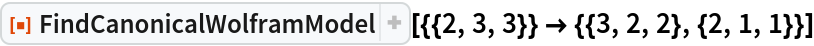Out=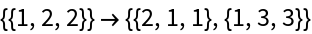Rename nodes and re-sort rules to find a canonical form:

 In:=Out=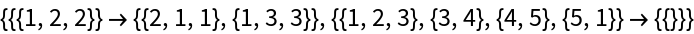A slightly more complicated case:

 In:=Out=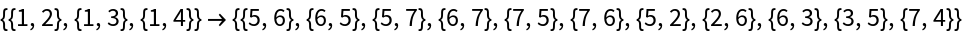### Scope (2)

FindCanonicalWolframModel can handle any names for elements:

 In:=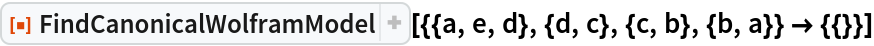Out=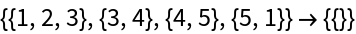In:=In:=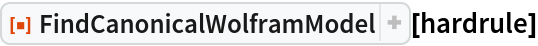Out=In:=In:=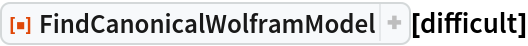Out=Some random lists of tuples that should have the same canonicalization:

 In:=In:=Out=The canonical forms are all identical:

 In:=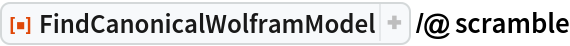Out=## Version History

• 4.0.0 – 17 February 2020
• 3.0.0 – 19 December 2019
• 2.0.0 – 13 December 2019
• 1.0.0 – 04 December 2019